# Zeta Converter

Description of the Zeta Converter component in Schematic Editor

A block diagram and input parameters for the Zeta Converter switching block are given in Table 1

Table 1. The Zeta Converter component in the HIL Toolbox
component component dialog window component parameters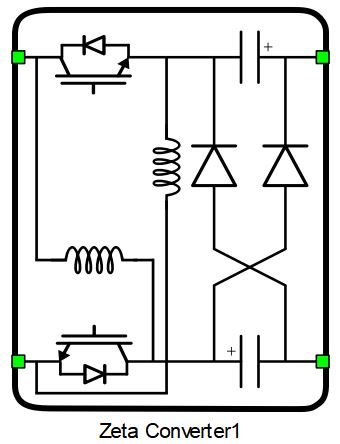• Property tabs
• General
• Electrical
Weight = 1 or 2

## Component dialogue box and parameters

The Zeta Converter component dialogue box consists of two tabs:
1. The General tab contains control-related properties. A detailed description can be seen in Control options.
2. Electrical tab parameters are shown in Table 2.
Table 2. Electrical tab properties
Property Code name Description
Inductance type ind_type Chooses between the inductor and the switched inductor
Double switch/inductor dbl_ind Enables the use of two equal inductances, each with a corresponding controllable switch. More details in Converter configurations section.
Inductance inductance Value of inductance of a single inductor. [H]
Initial current init_current Initial current of the inductors. [A]
Capacitance capacitance Value of capacitance of a single capacitor. [F]
Hybrid-switched capacitor hybrid_cap Enables the use of two equal capacitors, along with two diodes instead of one. More details in Converter configurations section.
Capacitor's ESR esr Equivalent series resistance of the capacitors. [Ω]
Initial voltage init_voltage Initial voltage of the capacitors. [V]

## Control options

Selecting Digital inputs as the Control parameter enables assigning gate drive inputs to any of the digital input pins (from 1 to 32(64)). For example, if S1 is assigned to 1, the digital input pin 1 will be routed to the S1 switch gate drive. In addition, the gate_logic parameter selects either active high (High-level input voltage VIH turns on the switch), or active low (Low-level input voltage VIL turns on the switch) gate drive logic, depending on the external controller design.

Selecting Internal modulator as the Control parameter, enables use of the internal PWM modulator for driving S1 switch instead of the digital input pins. In this configuration, two additional component inputs will be present. En input is used to enable/disable the internal PWM modulator, while In is used as a reference signal input.

Selecting Model, as the Control parameter, enables setting the IGBTs' gate drive signals directly from the signal processing model. The input pin gates appears on the component and requires a scalar input of gate drive signal for S1. When controlled from the model, logic is always active high.

## Converter configurations

The Zeta Converter component supports multiple modifications to its topology. For further details, see Schematic block diagrams of converter configurations. Modifications that can be done are based on the following three principles:
1. Inductor modification: The idea is to use a so-called switched inductor configuration instead of a single inductor. In steady state operation, when the controllable switch is in 'ON' state, diodes Dp1 and Dp2 are conducting, meaning that the inductors L1 and L2 are charged while connected in parallel. When the controllable switch is in 'OFF' state, only the Ds diode is conducting, thus connecting these inductors in series during discharge. For this reason, it is necessary to use the inductors of same value. This means that it is possible to obtain a larger voltage gain, since the inductance is 'easier to charge and harder to discharge'.Figure 1. Switched inductor
2. Capacitor modification: Similarly to the switched inductor, it is possible to use two capacitors, here refered to as a 'hybrid-switched capacitor', which further increases the voltage step-up ability of the converter.
3. It is possible to use two inductances with corresponding switches. This way, two inductances are charged while in a parallel connection and discharged while in a series connection. The two switches are controlled simultaneously.
Available converter configurations are given in Table 3. Their schematic diagrams can be seen in Schematic block diagrams of converter configurations. The input to output voltage ratio formula is derived under the assumptions of continuous current conduction mode and constant capacitor voltage, with D representing the duty cycle of controllable switch(es). The output LC filter should be connected externally for each of the configurations in order for the converter to be functional.
Table 3. Converter configurations
Property value Vout/Vin Configuration name
Inductance type Double switch/inductor Use hybrid switched capacitor Input to output voltage ratio of the converter Name of the configuration variant that is configured with each set of property values
Inductor False False
$\frac{D}{1-D}$
Single inductor
Inductor False True
$\frac{2D}{1-D}$
Single inductor with hybrid-switched capacitor
Inductor True False
$\frac{2D}{1-D}$
Double inductor
Inductor True True
$\frac{3D+1}{1-D}$
Double inductor with hybrid-switched capacitor
Switched inductor False False $\frac{D\left(D+1\right)}{1-D}$ Switched inductor
Switched inductor False True $\frac{D\left(D+3\right)}{1-D}$ Switched inductor with hybrid-switched capacitor
Switched inductor True False $\frac{2D\left(D+1\right)}{1-D}$ Double switched inductor
Switched inductor True True $\frac{{2D}^{2}+5D+1}{1-D}$ Double switched inductor with hybrid-switched capacitor

## Schematic block diagrams of converter configurations

Configurations that utilize a double inductor contain two switches. Since these switches are controlled simultaneously, only one switching signal is used. Therefore, the switch designated as 'S1' in the component parameters can represent one or two controllable switches, depending on how many are included.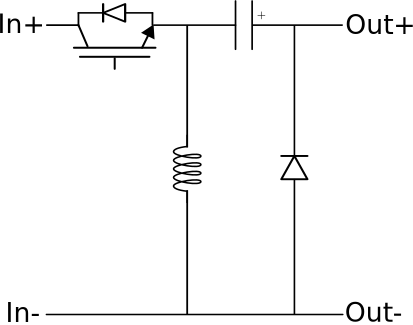Figure 2. Single inductor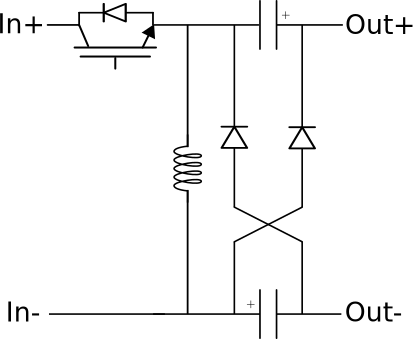Figure 3. Single inductor with hybrid-switched capacitor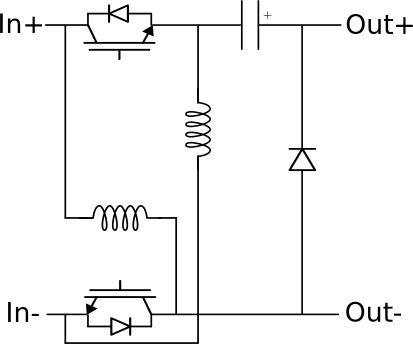Figure 4. Double inductor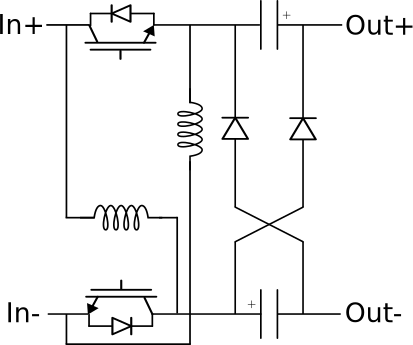Figure 5. Double inductor with hybrid-switched capacitor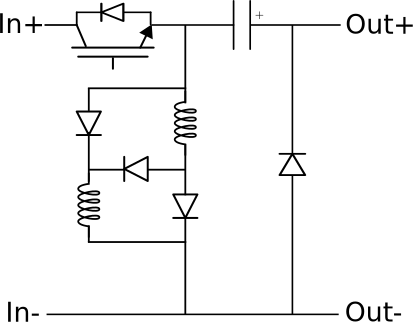Figure 6. Switched inductor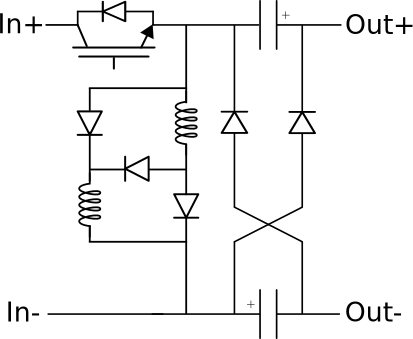Figure 7. Switched inductor with hybrid-switched capacitor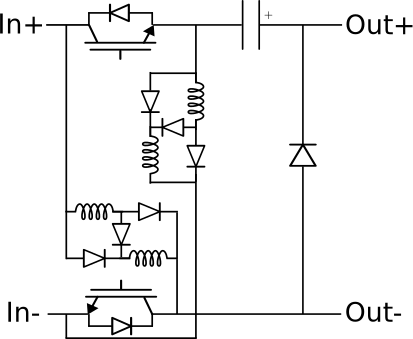Figure 8. Double switched inductor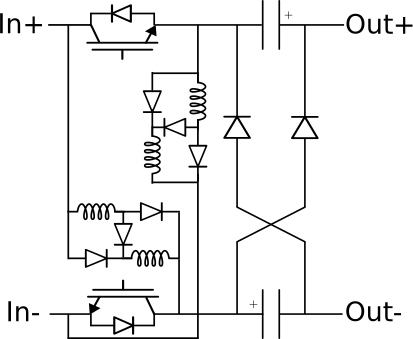Figure 9. Double switched inductor with hybrid-switched capacitor

## Digital Alias

If a converter is controlled by digital inputs, an alias for every digital input used by the converter will be created. Digital input aliases will be available under the Digital inputs list alongside existing Digital input signals. The alias will be shown as Converter_name.Switch_name, where Converter_name is name of the converter component and Switch_name is name of the controllable switch in the converter.

## References

1. B. Axelrod, Y. Berkovich and A. Ioinovici, 'Hybrid Switched - Capacitor Ćuk/Zeta/SEPIC Converters in Step-up Mode', 2005 IEEE International Symposium on Circuits and Systems, May 2005.
2. M. S. Bhaskar et al., "A New Hybrid Zeta-Boost Converter With Active Quad Switched Inductor for High Voltage Gain," in IEEE Access, vol. 9, pp. 20022-20034, 2021, doi: 10.1109/ACCESS.2021.3054393.